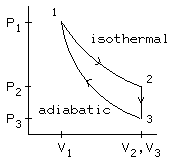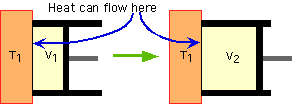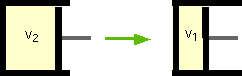Physics 213
Homework 2
Heat Capacity, Isothermal and Adiabatic Work, Heat Cycle

 Home Homework 1 Homework 2 Homework 3 Homework 4 Homework 5 Homework 6 About Us problem 1 HEAT CAPACITY OF AIR Given: p0 A sealed room, x meters on each side, filled with N2 (whose molecular weight = 28g/mol) Find: Heat required to raise the temp from T1ºC to T2ºC of N2 Solution: Use pV=NkT to find N, using p0 as the pressure, T1 as the temperature, and x^3 as the volume. Substitute that N value into the formula U = (alpha)Nk(deltaT) to get the answer. A Given: The same thing, except this time the air is 78.5% N2 and 21.5% O2 (molecular weight = 32g/mol) by volume. Find: Heat required to raise the temp from T1ºC to T2ºC of N2 Solution: The same as part A because the heat isn’t dependent on the composition of the air, note that you didn’t use the molecular weight of N2 in part A. B Given: Same Find: Heat Capacity Solution: C = Q/(deltaT), where Q is your answer from part A C

 problem 2 ISOTHERMAL WORK ON A GAS Given: X mols of nitrogen at a temp T1 in a volume Vi that is later compressed to Vf Find: Initial and Final Pressure Solution: Use pV=nRT twice, once for each volume A Given: The same thing Find: The work done on the nitrogen Solution: Wby = nRT ln (Vf/Vi). Watch the units. 1 L = .001m^3 B Given: Same Find: How much heat flows from the nitrogen into the environment Solution: deltaU = Wby - Q For isothermal processes, deltaU equals zero. Isothermal means there's no change in temp, and remember that deltaU = (alpha)NkT = (alpha)nRT. U is dependent on what kind of particles you're talking about (alpha = 3/2 or 5/2 etc) and temperature, so if there's no change in temp, there's no change in U. Thus that equation basically becomes Q = Wby. This is what you found in part B. C

 problem 3 ADIABATIC WORK ON A GAS Given: X mols of nitrogen at a temp T1 in a volume Vi that is later compressed to Vf Find: Initial and Final Pressure Solution: To get the initial pressure, use PV = nRT. To get the final pressure, use P1V1^(gamma) =P2V2^(gamma) where gamma = (alpha + 1)/alpha. Alpha in this case is 5/2 because nitrogen is diatomic, meaning it has 5 degreees of freedom (it can move in the directions of the x/y/z axes, and can spin in two ways), each degree being associated with .5kT. A Given: Same Find: Final temp (absolute) Solution: Use V1T1^(alpha) =V2T2^(alpha) B Given: Same Find: Work done on the nitrogen (in Joules) Solution: Won = -Wby = -(alpha)nR(T1-T2) If you haven't figured it out already, you better know which formulas on the equation sheet apply to which kind of process. C

 problem 4 HEAT CYCLE Given: A fixed quantity of an ideal monatomic gas (gamma = 5/3) is being taken around the cycle shown in the p-V diagram. p1 V1 T1 V2 = V 3 Know this picture. Understand it. And not just some half assed "I know what the picture looks like," you need to understand ALL of it: how to solve for values given values in other stages, which processes are reversible, how much work is done by each stage (by the way, you don't necessarily have to calculate values for that, you can look at the picture). And that's just to name a few things. We can almost guarantee that this kind of problem will come back and bite you in the ass on an exam.Here's what's happening: During the isothermal process, the gas expands while in contact with a large heat reservoir, so its temperature remains constant (T2 = T1).During the constant volume (isochoric) process, the gas is cooled from T2 to T3 by putting it in contact with a reservoir at temperature T3 . (This is an irreversible process.)During the adiabatic process, the gas is compressed while isolated from outside heat sources, so no heat flows. Work is done on the gas to compress it. The temperature rises from T3 to T1 , so the gas has returned to its original state.Find: What are the state 2 and 3 pressures Solution: The first step is isothermal, so keeping in mind temp stays the same, P1V1 = nRT = P2V2. You're given P1, V1, and V2, so just use P1V1=P2V2 to solve for P2. To find the pressure at stage 3, use the fact 1 -> 3 is an adiabatic process, so use P1V1^(gamma) =P3V3^(gamma) where gamma = 5/3, because it's a monatomic gas. A Given: The same thing, plus some extra stuff - In the following questions: Heat flow into the system, work done by the system, an increase in the internal energy of the system should be reported as positive . Heat flow out of the system, work done on the system, a decrease in the internal energy of the system should be reported as negative .   Find: Work done by this system and the heat flow into the system in the transition from state 1 to state 2 Solution: Work = nRT ln (Vf/Vi). As mentioned earlier, deltaU = 0 for isothermal processes (U= (alpha)nRT and there's no change in temp), so Q = Wby aka enter the same value for Q as you did for the work. B Given: Same Find: The same thing as part B but with the transition from state 2 to state 3 Solution: In an isochoric process there isn't a change in volume, so when looking at a graph it's a vertical line. Something important to note here, Work = integral of pdV, so think back to the days of calculus and realize that Work would also be the area under the pV curve. Isochoric pV curves are vertical, so there is no area under the curve, meaning no work is done. Thus, delta U = Q - Wby becomes deltaU = Q. U = (alpha)nRT = (alpha)pV right? So deltaU = (alpha)(deltaP)(V). You're given a V, you know the alpha = 3/2 because it's a monatomic gas (particles move in the x, y, and z directions), and you have both p values. So solve for U already. C Given: Same Find: Work done by this system and the change in the internal energy of this system in the transition from state 3 to state 1 Solution: 3 -> 1 is an adiabatic, so there's no change in Q. The equation deltaU = Q - Wby can then be simplified to deltaU = -Wby. deltaU = (alpha)nR(deltaT) = (alpha)(P3V3 - P1V1). You'll probably get a positive number for the deltaU, so just make it positive to get the Wby. D

 Got comments? Corrections? Or even better, donations? You can try us at LexiconIndustries@gmail.com, but just to warn you, we might not be home.